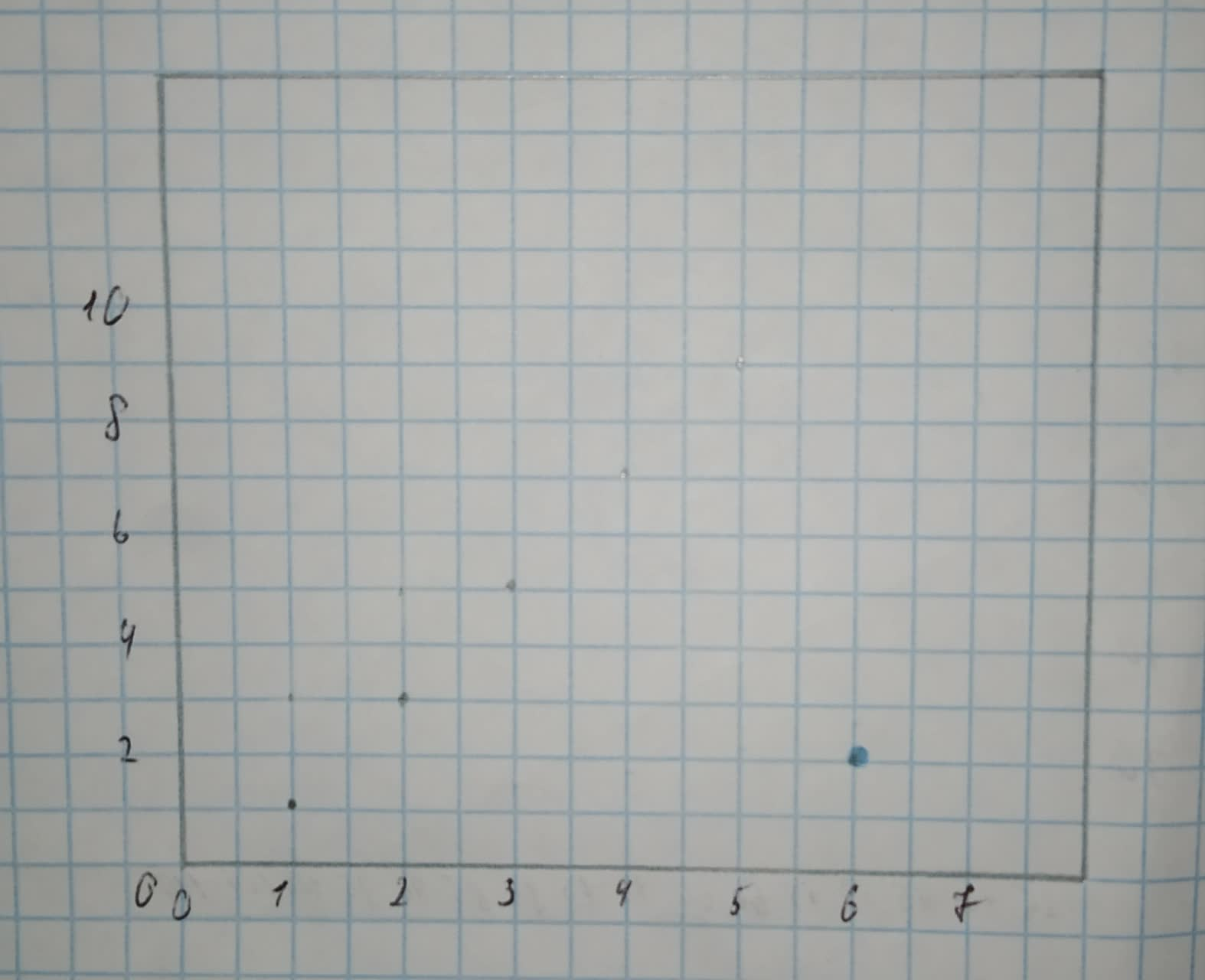he298c

2020-11-05

Sketch a scatterplot in which the presence of an outlier decreases the observed correlation between the response and explanatory variables. Indicate on your plot which point is the outlier.Malena

Step 1
Let the following data be the initial dataset (without any outliers):
[x 1 2 3 4 5 y 1 3 5 7 9]
Since all points lie on the line $\left[y=2x-1\right]$, the correlation between those two variables is $\left[r=1\right]$ (there is a perfect relationship between the two variables, since there exists a line that passes through all the points, and also, the slope of this line is $\left[{b}_{1}=2>0\right]$ so the association between the two variables is positive which implies that the correlation is also positive).
Let's add the point (6,2) to our initial dataset, so that the data is:
[x 1 2 3 4 5 6 y 1 3 5 7 9 2]
The outlier that we've just added clearly doesn’t belong to the line $\left[y=2x-1\right]$
since $\left[2\ne q2\cdot 6-1=11\right]$.
Therefore, we lost the perfect relationship between the two variables, so the correlation coefficient decreased and the new correlation coefficient is $\left[r<1\right]$.
Here is the scatterplot of the given data (outlier is coloured in blue):Do you have a similar question?